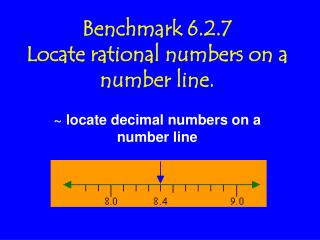DownloadDownload PresentationBenchmark 6.2.7 Locate rational numbers on a number line.

# Benchmark 6.2.7 Locate rational numbers on a number line.

Télécharger la présentation## Benchmark 6.2.7 Locate rational numbers on a number line.

- - - - - - - - - - - - - - - - - - - - - - - - - - - E N D - - - - - - - - - - - - - - - - - - - - - - - - - - -
##### Presentation Transcript

1. Benchmark 6.2.7Locate rational numbers on a number line. ~ locate decimal numbers on a number line

2. Who needs to locate decimal numbers on a number line?

3. Machinists and other skilled workers use decimal rulers to make measurements! Some use Vernier Calipers

4. Some use hundredth scales. This makes their measurements very accurate.

5. You can also put decimals on a number line to compare and order them. DecimalsAs you read a typical number linefrom left to right, the decimals will become greater, and get closer to the next whole number.

6. What if two numbers have different place values? Example: 0.7 and 0.53

7. The easiest way to fix that is to add zeros to make your decimal numbers have the same place value. Remember! Adding on zeros will NOT change the value of your decimal number!

8. Add a 0 to 0.7 = 0.70.70 is larger than .53, so it will come after 0.53 on the number line. 0.53 0.70

9. What number does point N represent on the number line below? Remember to look at the intervals! Correct! 2.6

10. What numbers are represented by the letters A, B, and C? A B C A = 4.81 B = 4.93 C = 5.04

11. Now, let’s do some independent practice! Turn to page 2 in your handout!